## Poll

 I love math!21 votes (46.66%) Math is great.14 votes (31.11%) My religion is mathology.6 votes (13.33%) Women didn't speak to me until I was 30.2 votes (4.44%) Total eclipse reminder -- 04/08/202412 votes (26.66%) I steal cutlery from restaurants.3 votes (6.66%) I should just say what's on my mind.6 votes (13.33%) Who makes up these awful names for pandas?5 votes (11.11%) I like to touch my face.12 votes (26.66%) Pork chops and apple sauce.10 votes (22.22%)

45 members have voted

GialmereJoined: Nov 26, 2018
• Posts: 2800
May 19th, 2020 at 11:32:00 AM permalink
Heh. The mathematics of pancakes eh? I haven't been to an IHOP for a long time. Now I want to go just to see how difficult it is to slice a flapjack horizontally. I think it's possible.

Crepes, on the other hand, are whole different level of difficulty.
Have you tried 22 tonight? I said 22.
GialmereJoined: Nov 26, 2018
• Posts: 2800
May 20th, 2020 at 7:42:46 AM permalink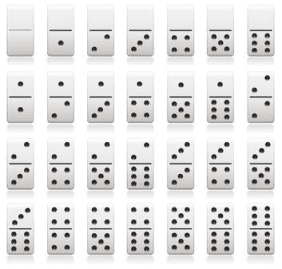Woohoo! Your local casino just reopened. You agree to meet your friend there to spend the day.

When you arrive, you find your friend playing a new game called Casino Dominoes which you remember reading about at WOO. Your friend wants to play one more hand before you go have drinks so you slap some money down on the table. Unfortunately, you not only don't score any points, none of your three dominoes even have a matching number to connect to the community domino.

You head to the bar. While you friend is getting drinks you contemplate the odds of dominoes. What is the probability that two dominoes drawn randomly from a single standard set will have a connecting match (will allow concatenation)?
Have you tried 22 tonight? I said 22.
ThatDonGuyJoined: Jun 22, 2011
• Posts: 5947
Thanks for this post from:May 20th, 2020 at 8:23:13 AM permalink

Of the 28 dominoes, seven are doubles (double-blank through double-6), and 21 are two different numbers.
If you draw a double, 6 of the remaining 27 dominoes will match it (e.g. if you draw a 1-1, it matches 1-blank, 1-2, 1-3, 1-4, 1-5, and 1-6).
If you draw a non-double, 12 of the remaining 27 dominoes will match it (e.g. if you draw a 1-2, it matches 1-blank, 1-1, 1-3, 1-4, 1-5, 1-6, 2-blank, 2-2, 2-3, 2-4, 2-5, and 2-6).

The probability of a match is 1/4 x 2/9 + 3/4 x 4/9 = 7/18.

GialmereJoined: Nov 26, 2018
• Posts: 2800
May 20th, 2020 at 8:33:58 AM permalink
Quote: ThatDonGuy

Of the 28 dominoes, seven are doubles (double-blank through double-6), and 21 are two different numbers.
If you draw a double, 6 of the remaining 27 dominoes will match it (e.g. if you draw a 1-1, it matches 1-blank, 1-2, 1-3, 1-4, 1-5, and 1-6).
If you draw a non-double, 12 of the remaining 27 dominoes will match it (e.g. if you draw a 1-2, it matches 1-blank, 1-1, 1-3, 1-4, 1-5, 1-6, 2-blank, 2-2, 2-3, 2-4, 2-5, and 2-6).

The probability of a match is 1/4 x 2/9 + 3/4 x 4/9 = 7/18.

Correct!

Small wonder the lowest payout for Casino Dominoes is 2:1.
-------------------------------

My friend told me a joke about dominoes.

It had a really long set up, but in the end it fell flat.
Have you tried 22 tonight? I said 22.
Ace2Joined: Oct 2, 2017
• Posts: 2664
May 20th, 2020 at 9:50:07 PM permalink
Two craps players (A and B) decide to make a side wager. A only plays the passline. B only plays don't pass. Starting with the next comeout roll, the first player to get 10 wins is the winner of the side wager. Every bet resolved is either a win A or a win for B, except for a 12 on a comeout roll, which is a win for neither

What are A's chances of winning the side wager ?

Answers should be expressed a rational number
Last edited by: Ace2 on May 20, 2020
It�s all about making that GTA
WizardJoined: Oct 14, 2009
• Posts: 25957
May 20th, 2020 at 10:44:16 PM permalink
Quote: ThatDonGuy

It's a straightforward Fibonacci sequence that starts with 0
0, 1, 1, 2, 3, 5, 8, 13, 21, 34, 55, 89, 144, 233, 377, 610, 987, 1597, 2584, 4181, 6765

There are 6765 mature and 4181 immature microbes = 10,946 total

Correct!
�Extraordinary claims require extraordinary evidence.� -- Carl Sagan
ChesterDogJoined: Jul 26, 2010
• Posts: 1369
May 20th, 2020 at 11:42:39 PM permalink
Quote: Ace2

Two craps players (A and B) decide to make a side wager. A only plays the passline. B only plays don't pass. Starting with the next comeout roll, the first player to get 10 wins is the winner of the side wager. Every bet resolved is either a win A or a win for B, except for a 12 on a comeout roll, which is a win for neither

What are A's chances of winning the side wager ?

Answers should be expressed a rational number

Just kidding with 0.52!

I get 2419531625796339034080102292958091287906159377026607445180416 / 4611277450446459784127710060894226116943173110485076904296875

Please explain in detail how you did it?
ThatDonGuyJoined: Jun 22, 2011
• Posts: 5947
May 21st, 2020 at 8:01:19 AM permalink
Quote: ChesterDog

Just kidding with 0.52!

I get 2419531625796339034080102292958091287906159377026607445180416 / 4611277450446459784127710060894226116943173110485076904296875

Please explain in detail how you did it?

I get the same answer ChesterDog does.

Ignoring comeout rolls of 12, Pass wins 976/1925 of the time, and Don't Pass wins 949/1925 of the time.

Let p = 976/1925 and q = 949/1925
There is one way of 10 passes in a row; this has probability p10.
There are 10C1 ways of 9 passes and 1 don't pass, in some order, followed by a pass; each has probability p10 q.
There are 11C2 ways of 9 passes and 2 don't passes, in some order, followed by a pass; each has probability p10 q2.
...and so on, through
There are 18C9 ways of 9 passes and 9 don't passes, in some order, followed by a pass; each has probability p10 q9.

How did I calculate the sum and then reduce the result to a fraction of relatively prime terms? That's what the BigInteger class in Microsoft .NET is for.

Ace2Joined: Oct 2, 2017
• Posts: 2664
May 21st, 2020 at 9:53:39 AM permalink
Quote: ChesterDog

Just kidding with 0.52!

I get 2419531625796339034080102292958091287906159377026607445180416 / 4611277450446459784127710060894226116943173110485076904296875

Please explain in detail how you did it?

Correct!

It�s all about making that GTA
ChesterDogJoined: Jul 26, 2010
• Posts: 1369
May 21st, 2020 at 11:40:05 AM permalink
Quote: Ace2

Quote: ChesterDog

Just kidding with 0.52!

I get 2419531625796339034080102292958091287906159377026607445180416 / 4611277450446459784127710060894226116943173110485076904296875

Please explain in detail how you did it?

Correct!

The probability of �pass� winning is:
(1/36)(0)+(2/36)(0)+(3/36)(3/9)+(4/36)(4/10)+(5/36)(5/11)+(6/36)(1)
+(5/36)(5/11)+(4/36)(4/10)+(3/36)(3/9)+(2/36)(1)+(1/36)(0)

And the probability of �don�t pass� winning is:

(1/36)(1)+(2/36)(1)+(3/36)(6/9)+(4/36)(6/10)+(5/36)(6/11)+(6/36)(0)
+(5/36)(6/11)+(4/36)(6/10)+(3/36)(6/9)+(2/36)(0)+(1/36)(0)

To evaluate these expressions precisely, I input them into the online calculator WolframAlpha. To avoid typos, the Excel function �concatenate� is used to prepare the expressions.

When twelve is rolled, neither pass nor don�t pass wins, so we will ignore twelves. Let A and B stand for the probabilities of pass winning and don�t pass winning, respectively, each divided by the quantity one minus the probability of 12.

Then:
A = 976/1925; B = 949/1925

For player A to win ten passes before B wins ten don�t passes, A must win nine, while B may win nine or fewer. And then A must win for his tenth time.

The probability that A wins nine while B wins �k� times is A9Bkcombin(9+k,k). For example, if k = 7, then this term would be (976/1925)9(949/1925)7(11,440).

We add the terms for k = 0 to k = 9 and multiply by A to get the final answer.

Below is WolframAlpha�s input:
(976/1925)^10(1(949/1925)^0+10(949/1925)^1+55(949/1925)^2
+220(949/1925)^3+715(949/1925)^4+2002(949/1925)^5+5005(949/1925)^6
+11440(949/1925)^7+24310(949/1925)^8+48620(949/1925)^9)

And WolframAlpha gives the answer precisely as the ratio of two long integers.

ThatDonGuy used the same method but without WolframAlpha.
Ace2Joined: Oct 2, 2017
• Posts: 2664
May 21st, 2020 at 8:22:21 PM permalink
I used Poisson. Take the integral over all time of:

(ax)^9*e^(-ax)/9!*e^(-bx)*((bx)^9/9!+(bx)^8/8!+(bx)^7/7!+(bx)^6/6!+(bx)^5/5!+(bx)^4/4!+(bx)^3/3!+(bx)^2/2+(bx)+1)*a dx

Where a = 976/1925 and b = 949/1925

Which comes to:

2419531625796339034080102292958091287906159377026607445180416 / 4611277450446459784127710060894226116943173110485076904296875 =~ 0.52
It�s all about making that GTA
GialmereJoined: Nov 26, 2018
• Posts: 2800
May 22nd, 2020 at 8:05:54 AM permalink
BOB x BOB = MARLEY

For no particular reason, this easy math puzzle celebrates Bob Marley. Ya mon.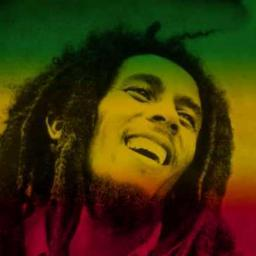In the multiplication problem below, each digit has been replaced by a letter. Can you determine the value of each letter?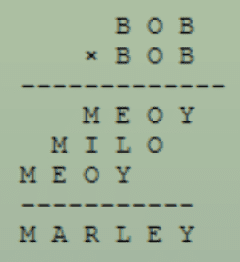B = ?
O = ?
M = ?
A = ?
R = ?
L = ?
E = ?
Y = ?
I = ?

Have you tried 22 tonight? I said 22.
ThatDonGuyJoined: Jun 22, 2011
• Posts: 5947
Thanks for this post from:May 22nd, 2020 at 10:17:39 AM permalink

Note that neither B nor O are 0 or 1, as none of the intermediate lines is 0 or BOB.
Also, B cannot be 5 or 6, as B x B would end in B, but B x B ends in Y.
O x B ends in O
The (O,B) pairs where O and B are distinct digits from 2 to 9 and this is true are (2,6), (4,6), (5,3), (5,7), (5,9), and (8,6).
Since B is not 6, O must be 5.
BOB x B and BOB x O are both four-digit numbers where the first digit is the same.
757 x 5 = 3785, but 757 x 7 = 5299, so (5,7) is incorrect.
959 x 5 = 4795, but 959 x 9 = 8631, so (5,9) is incorrect.
Therefore, B = 3, and the product is 353 x 353; MEOY = 1059, MILO = 1765, and MARLEY = 124,609.

B,O,M,A,R,L,E,Y are 3,5,1,2,4,6,0,9 (and I is 7)

GialmereJoined: Nov 26, 2018
• Posts: 2800
May 22nd, 2020 at 4:22:53 PM permalink
Quote: ThatDonGuy

Note that neither B nor O are 0 or 1, as none of the intermediate lines is 0 or BOB.
Also, B cannot be 5 or 6, as B x B would end in B, but B x B ends in Y.
O x B ends in O
The (O,B) pairs where O and B are distinct digits from 2 to 9 and this is true are (2,6), (4,6), (5,3), (5,7), (5,9), and (8,6).
Since B is not 6, O must be 5.
BOB x B and BOB x O are both four-digit numbers where the first digit is the same.
757 x 5 = 3785, but 757 x 7 = 5299, so (5,7) is incorrect.
959 x 5 = 4795, but 959 x 9 = 8631, so (5,9) is incorrect.
Therefore, B = 3, and the product is 353 x 353; MEOY = 1059, MILO = 1765, and MARLEY = 124,609.

B,O,M,A,R,L,E,Y are 3,5,1,2,4,6,0,9 (and I is 7)Correct!

Good job mon! You be jammin!
--------------------------

Some historians speculate that instead of cancer, Bob Marley died of an acute case of ganjarea.
Have you tried 22 tonight? I said 22.
gamerfreakJoined: Dec 28, 2014
• Posts: 3540
May 23rd, 2020 at 9:06:01 AM permalink
Probably too easy, but I like the question.

What is the probability of a broken clock being correct?
Ace2Joined: Oct 2, 2017
• Posts: 2664
May 23rd, 2020 at 9:10:12 AM permalink
Quote: gamerfreak

Probably too easy, but I like the question.

What is the probability of a broken clock being correct?

Zero. The level of precision is infinite. Like the probability of two people being exactly the same height
It�s all about making that GTA
unJonJoined: Jul 1, 2018
• Posts: 4344
May 23rd, 2020 at 9:13:50 AM permalink
Quote: Ace2

Zero. The level of precision is infinite. Like the probability of two people being exactly the same height

And yet it happens twice a day.
The race is not always to the swift, nor the battle to the strong; but that is the way to bet.
EdCollinsJoined: Oct 21, 2011
• Posts: 1739
May 23rd, 2020 at 9:40:44 AM permalink
Quote: gamerfreak

What is the probability of a broken clock being correct?

24 hours x 60 minutes x 60 seconds = 86,400 seconds each day.

86,400 / 2 = 0.0000231481481481481
CrystalMathJoined: May 10, 2011
• Posts: 1909
May 23rd, 2020 at 9:52:16 AM permalink
While a broken clock is correct twice a day, most working clocks are always wrong.
I heart Crystal Math.
GialmereJoined: Nov 26, 2018
• Posts: 2800
May 24th, 2020 at 4:17:43 AM permalink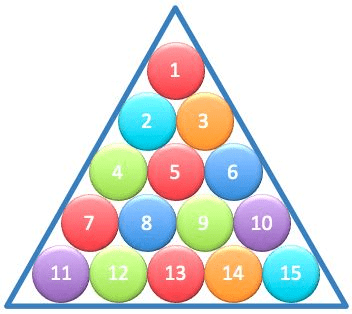Meanwhile, at the pool hall, you place 15 balls into a snug, exactly fitting triangle rack...

You remove one of the balls and notice that all the balls left in the rack are still immobile and locked in place. How many balls can you remove from the rack and still have all the balls remaining stay (at least theoretically) locked in place.

When you set up to re-rack the balls, you're stunned to see that the side lengths of the triangle rack have all magically decreased by 20%. (This is what happens when you shoot pool after visiting the NuWu Marketplace.) How many normal size balls will fit into this smaller rack?Have you tried 22 tonight? I said 22.
ThatDonGuyJoined: Jun 22, 2011
• Posts: 5947
Thanks for this post from:May 24th, 2020 at 8:30:13 AM permalink

#1: Note that you cannot remove two balls that touch each other without removing the ball(s) that touch both of them, as those balls would then be able to move.

The best I can come up with is six - remove 1, 4, 6, 11, 13, and 15

#2: I can fit seven, but I have a feeling there's a way to fit eight.
Leave 1, 2, 3, 4 where they are; move 5 so that it touches 4 and the bottom of the rack; move 6 so that it touches 3 and 5; 7 should fit in the lower right corner.

GialmereJoined: Nov 26, 2018
• Posts: 2800
May 25th, 2020 at 11:56:25 AM permalink
Quote: ThatDonGuy

#1: Note that you cannot remove two balls that touch each other without removing the ball(s) that touch both of them, as those balls would then be able to move.

The best I can come up with is six - remove 1, 4, 6, 11, 13, and 15

#2: I can fit seven, but I have a feeling there's a way to fit eight.
Leave 1, 2, 3, 4 where they are; move 5 so that it touches 4 and the bottom of the rack; move 6 so that it touches 3 and 5; 7 should fit in the lower right corner.

Correct!

6 it is.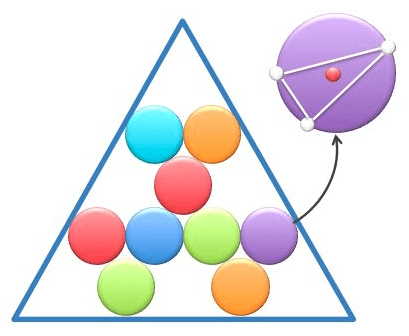A ball cannot move if its center is in the polygon based on its support points.

7 is correct...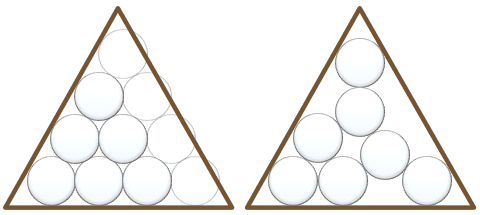Have you tried 22 tonight? I said 22.
GialmereJoined: Nov 26, 2018
• Posts: 2800
May 25th, 2020 at 12:25:29 PM permalinkYou decide to mess around with a pair of fair dice and see if you can come up with a new casino dice game...

For your first mechanic, you try rolling one die first and then rolling the second die. What is the probability that the second number is greater than the first?

The second mechanic you try consists of rolling both dice and then multiplying the numbers rolled. What product is most likely to occur?
Have you tried 22 tonight? I said 22.
rsactuaryJoined: Sep 6, 2014
• Posts: 2297
Thanks for this post from:May 25th, 2020 at 1:45:08 PM permalink
Problem 1: (5+4+3+2+1+0)/36 = 15/36

Problem 2: 6 and 12 appears 4 times. Had to do this by brute force. Interested if someone has a more mathematical way. ETA: In all fairness, I added "6" after reading unJon's answer.
Last edited by: rsactuary on May 25, 2020
unJonJoined: Jul 1, 2018
• Posts: 4344
Thanks for this post from:May 25th, 2020 at 1:50:57 PM permalink
Quote: GialmereYou decide to mess around with a pair of fair dice and see if you can come up with a new casino dice game...

For your first mechanic, you try rolling one die first and then rolling the second die. What is the probability that the second number is greater than the first?

The second mechanic you try consists of rolling both dice and then multiplying the numbers rolled. What product is most likely to occur?

6/36 of the time the two dice are tied. Of the remaining 30 times, half the time the second die is higher. So 15/36 or about 41.67%

I did this in Excel and got that 6 and 12 are the most likely products with 4 instances each of the 36 possibilities
The race is not always to the swift, nor the battle to the strong; but that is the way to bet.
GialmereJoined: Nov 26, 2018
• Posts: 2800
May 25th, 2020 at 11:38:21 PM permalink
Quote: rsactuary

Problem 1: (5+4+3+2+1+0)/36 = 15/36

Problem 2: 6 and 12 appears 4 times. Had to do this by brute force. Interested if someone has a more mathematical way. ETA: In all fairness, I added "6" after reading unJon's answer.

Quote: unJon

6/36 of the time the two dice are tied. Of the remaining 30 times, half the time the second die is higher. So 15/36 or about 41.67%

I did this in Excel and got that 6 and 12 are the most likely products with 4 instances each of the 36 possibilities

Correct!

Note for problem #1 the second number MUST be greater and therefore the odds are against it.

For problem #2 yes, 6 and 12 are most likely. Perhaps a baccarat style game (with 6 and 12 acting as player and banker wagers) along with side bets while waiting for a winner.

[To be continued...]
Have you tried 22 tonight? I said 22.
GialmereJoined: Nov 26, 2018
• Posts: 2800
May 27th, 2020 at 8:01:01 AM permalink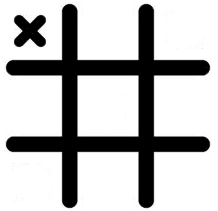You're messing around with a simple Tic-Tac-Toe computer program. You set it so that three Xs are randomly placed on an empty grid. Rounded to the nearest percentage point, what is the probability that the three Xs will form a "three in a row" pattern?

Bonus: What is the greatest number of Xs you can place on an empty grid without making a "three in a row" pattern?
Have you tried 22 tonight? I said 22.
JoemanJoined: Feb 21, 2014
• Posts: 2372
Thanks for this post from:May 27th, 2020 at 8:29:46 AM permalink
Quote: GialmereYou're messing around with a simple Tic-Tac-Toe computer program. You set it so that three Xs are randomly placed on an empty grid. Rounded to the nearest percentage point, what is the probability that the three Xs will form a "three in a row" pattern?

Bonus: What is the greatest number of Xs you can place on an empty grid without making a "three in a row" pattern?

To the nearest percent, I get 10%.

Since the odds of completing three-in-a-row are different, depending on where the first X is placed, let's look at the three distinct cases individually, and then sum the probabilities.

1) Start in a corner (4/9 chance): 6 of the 8 remaining boxes will facilitate the three-in-a-row. Two will not help. Only 1 of the 7 remaining boxes will complete the line. So, this probability is: 4/9 x 6/8 x 1/7 = 0.0476

2) Start in a mid-side box (4/9 chance): 4 of the 8 remaining boxes will facilitate the three-in-a-row, and only 1 of the 7 remaining boxes will complete the line. So, this probability is: 4/9 x 4/8 x 1/7 = 0.0317

3) Start in the center (1/9 chance): Any of the remaining boxes will facilitate the three-in-a-row, but only 1 of the 7 remaining boxes will complete the line. So, this probability is: 1/9 x 1 x 1/7 = 0.0159

Summing, you get 0.0952, which rounds to 10%.

6. If you leave a diagonal blank and put x's in the remaining boxes, you can get 6 in without making a 3-in-a-row.
"Dealer has 'rock'... Pay 'paper!'"
GialmereJoined: Nov 26, 2018
• Posts: 2800
May 27th, 2020 at 9:00:22 AM permalink
Quote: Joeman

To the nearest percent, I get 10%.

Since the odds of completing three-in-a-row are different, depending on where the first X is placed, let's look at the three distinct cases individually, and then sum the probabilities.

1) Start in a corner (4/9 chance): 6 of the 8 remaining boxes will facilitate the three-in-a-row. Two will not help. Only 1 of the 7 remaining boxes will complete the line. So, this probability is: 4/9 x 6/8 x 1/7 = 0.0476

2) Start in a mid-side box (4/9 chance): 4 of the 8 remaining boxes will facilitate the three-in-a-row, and only 1 of the 7 remaining boxes will complete the line. So, this probability is: 4/9 x 4/8 x 1/7 = 0.0317

3) Start in the center (1/9 chance): Any of the remaining boxes will facilitate the three-in-a-row, but only 1 of the 7 remaining boxes will complete the line. So, this probability is: 1/9 x 1 x 1/7 = 0.0159

Summing, you get 0.0952, which rounds to 10%.

6. If you leave a diagonal blank and put x's in the remaining boxes, you can get 6 in without making a 3-in-a-row.

Correct!

And impressively quick.
Have you tried 22 tonight? I said 22.
ThatDonGuyJoined: Jun 22, 2011
• Posts: 5947
Thanks for this post from:May 27th, 2020 at 10:07:29 AM permalink
Quote: GialmereYou're messing around with a simple Tic-Tac-Toe computer program. You set it so that three Xs are randomly placed on an empty grid. Rounded to the nearest percentage point, what is the probability that the three Xs will form a "three in a row" pattern?

Bonus: What is the greatest number of Xs you can place on an empty grid without making a "three in a row" pattern?

#1 - there are 9C3 = 84 ways to place the three Xs, of which eight (three across, three down, two diagonally) are three in a row, so the probability = 8/84 = 2/21, which is closest to 10%

#2 - by the Pigeonhole Principle, it is impossible to place seven Xs without at least one row (and at least one column, for that matter) having three Xs. As already pointed out, leaving either diagonal empty allows for 6 Xs without three in a row; since 6 is possible and 7 (or more) is not, 6 is the solution.

GialmereJoined: Nov 26, 2018
• Posts: 2800
May 27th, 2020 at 4:13:39 PM permalink
Quote: ThatDonGuy

#1 - there are 9C3 = 84 ways to place the three Xs, of which eight (three across, three down, two diagonally) are three in a row, so the probability = 8/84 = 2/21, which is closest to 10%

#2 - by the Pigeonhole Principle, it is impossible to place seven Xs without at least one row (and at least one column, for that matter) having three Xs. As already pointed out, leaving either diagonal empty allows for 6 Xs without three in a row; since 6 is possible and 7 (or more) is not, 6 is the solution.

Correct!

Very clever Mr. Bond.
Have you tried 22 tonight? I said 22.
GialmereJoined: Nov 26, 2018
• Posts: 2800
May 28th, 2020 at 7:28:49 AM permalink
Similar to the pancake problem...Two triangles form seven separate regions. What is the greatest number of such regions that can be formed by three triangles?
Have you tried 22 tonight? I said 22.
ThatDonGuyJoined: Jun 22, 2011
• Posts: 5947
May 28th, 2020 at 8:04:27 AM permalink
Quote: Gialmere

Two triangles form seven separate regions. What is the greatest number of such regions that can be formed by three triangles?

...16

Choose three points outside of any triangle - one just outside of where regions 1 and 2 meet, one just outside of where 3 and 4 meet, and one just outside of where 5 and 6 meet; call them A, B, and C, respectively.
AB goes through regions 2, 7, and 3, in that order.
BC goes through 4, 7, and 5, in that order.
CA goes through 6, 7, and 1, in that order.
Regions 1 through 6 are divided into 2 regions, and Region 7 is divided into 4.

unJonJoined: Jul 1, 2018
• Posts: 4344
May 28th, 2020 at 8:15:24 AM permalink
Quote: ThatDonGuy

Quote: Gialmere

Two triangles form seven separate regions. What is the greatest number of such regions that can be formed by three triangles?

...16

Choose three points outside of any triangle - one just outside of where regions 1 and 2 meet, one just outside of where 3 and 4 meet, and one just outside of where 5 and 6 meet; call them A, B, and C, respectively.
AB goes through regions 2, 7, and 3, in that order.
BC goes through 4, 7, and 5, in that order.
CA goes through 6, 7, and 1, in that order.
Regions 1 through 6 are divided into 2 regions, and Region 7 is divided into 4.

I get the same.
The race is not always to the swift, nor the battle to the strong; but that is the way to bet.
GialmereJoined: Nov 26, 2018
• Posts: 2800
May 28th, 2020 at 9:00:40 AM permalink
16 is incorrect.
Have you tried 22 tonight? I said 22.
charliepatrickJoined: Jun 17, 2011
• Posts: 2933
Thanks for this post from:May 28th, 2020 at 11:37:56 AM permalink
Just a trial and error I can get to 19.

If you look at the two triangles then, for arguments sake, you can draw a line that cuts through 1 and 2 (i.e. creates 2 more within those areas) and either forms a triangle outside and between 1 and 2 or inside the middle. So this creates three more areas.

Repeat for the other two pairs, this creates six more areas.

Also where the lines meet (outside the original triangles) there are three more areas.

In total this is 12 more areas, leading to a total of 19.
I think the earlier answers used a similar idea but forgot the lines formed another area whenever a pair of them met each other outside the original two triangles. Hence there are three more than the 16.
GialmereJoined: Nov 26, 2018
• Posts: 2800
May 28th, 2020 at 12:03:59 PM permalink
Quote: charliepatrick

Just a trial and error I can get to 19.

If you look at the two triangles then, for arguments sake, you can draw a line that cuts through 1 and 2 (i.e. creates 2 more within those areas) and either forms a triangle outside and between 1 and 2 or inside the middle. So this creates three more areas.

Repeat for the other two pairs, this creates six more areas.

Also where the lines meet (outside the original triangles) there are three more areas.

In total this is 12 more areas, leading to a total of 19.
I think the earlier answers used a similar idea but forgot the lines formed another area whenever a pair of them met each other outside the original two triangles. Hence there are three more than the 16.

Correct!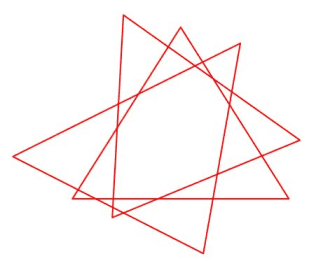Have you tried 22 tonight? I said 22.
GialmereJoined: Nov 26, 2018
• Posts: 2800
May 29th, 2020 at 7:46:00 AM permalinkWhile setting up a new account, you need to create a four digit PIN code. You decide to use four different numbers with each number smaller than the previous one in the sequence. How many code options do you have?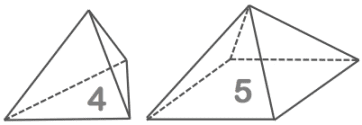A pyramid and a tetrahedron with edges of the same length are glued together on a triangular face. How many faces does the resulting solid have?
Have you tried 22 tonight? I said 22.
rsactuaryJoined: Sep 6, 2014
• Posts: 2297
May 29th, 2020 at 8:08:35 AM permalink
Puzzle 1: 125?
ThatDonGuyJoined: Jun 22, 2011
• Posts: 5947
Thanks for this post from:May 29th, 2020 at 8:26:41 AM permalink
Quote: GialmereWhile setting up a new account, you need to create a four digit PIN code. You decide to use four different numbers with each number smaller than the previous one in the sequence. How many code options do you have?A pyramid and a tetrahedron with edges of the same length are glued together on a triangular face. How many faces does the resulting solid have?

There are 10C4 = 210 sets of 4 different digits, and each set has exactly one way to arrange the digits in decreasing order, so the answer is 210.

I think it's 7 (5 + 4, minus 2 for the glued sides that are now part of the interior of the solid).
I thought that maybe two sides of the pyramid and two sides of the tetrahedron would form two sides of the new solid, but the bases of the two pyramid sides are parallel and the bases of the two tetrahedron sides aren't.

GialmereJoined: Nov 26, 2018
• Posts: 2800
May 29th, 2020 at 10:09:05 AM permalink
Quote: ThatDonGuy

There are 10C4 = 210 sets of 4 different digits, and each set has exactly one way to arrange the digits in decreasing order, so the answer is 210.

Correct!

Quote: ThatDonGuy

I think it's 7 (5 + 4, minus 2 for the glued sides that are now part of the interior of the solid).
I thought that maybe two sides of the pyramid and two sides of the tetrahedron would form two sides of the new solid, but the bases of the two pyramid sides are parallel and the bases of the two tetrahedron sides aren't.

7 is incorrect (but you're hot on the trail).
Have you tried 22 tonight? I said 22.
ThatDonGuyJoined: Jun 22, 2011
• Posts: 5947
Thanks for this post from:May 29th, 2020 at 10:38:02 AM permalink
Quote: Gialmere

Quote: ThatDonGuy

There are 10C4 = 210 sets of 4 different digits, and each set has exactly one way to arrange the digits in decreasing order, so the answer is 210.

Correct!

Quote: ThatDonGuy

I think it's 7 (5 + 4, minus 2 for the glued sides that are now part of the interior of the solid).
I thought that maybe two sides of the pyramid and two sides of the tetrahedron would form two sides of the new solid, but the bases of the two pyramid sides are parallel and the bases of the two tetrahedron sides aren't.

7 is incorrect (but you're hot on the trail).

I see now...the bases edges of the pyramid are parallel, but the faces aren't, and when the tetrahedron is attached, each of the two faces is in the same plane with a face of the tetrahedron, so there are only 5 faces in the result.

To be fair, I had heard of this before, which is why my original answer was a little hesitant.

This reminds me of another problem on an SAT that had an incorrect answer:

You have two coins that are tangent to each other. One has a diameter three times as large as the other. The smaller one is rolled around the larger one until it goes all the way around the larger one exactly once. How many revolutions of itself did the smaller coin make?
gordonm888Joined: Feb 18, 2015
• Posts: 4808
May 29th, 2020 at 10:43:28 AM permalink
Quote: ThatDonGuy

This reminds me of another problem on an SAT that had an incorrect answer:

You have two coins that are tangent to each other. One has a diameter three times as large as the other. The smaller one is rolled around the larger one until it goes all the way around the larger one exactly once. How many revolutions of itself did the smaller coin make?

4 revolutions

The multiple choice answers on this SAT question did not offer this correct answer. The testing company assumed that 3 rotations of the small circle around its own center was the correct answer.
So many better men, a few of them friends, are dead. And a thousand thousand slimy things live on, and so do I.
GialmereJoined: Nov 26, 2018
• Posts: 2800
May 29th, 2020 at 11:01:06 AM permalink
Quote: ThatDonGuy

I see now...the bases edges of the pyramid are parallel, but the faces aren't, and when the tetrahedron is attached, each of the two faces is in the same plane with a face of the tetrahedron, so there are only 5 faces in the result.

To be fair, I had heard of this before, which is why my original answer was a little hesitant.

Correct!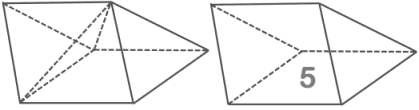It's hard to visualize in the mind but two pairs of adjacent faces in the combined solid are coplaner.
Last edited by: Gialmere on May 29, 2020
Have you tried 22 tonight? I said 22.
ThatDonGuyJoined: Jun 22, 2011
• Posts: 5947
May 29th, 2020 at 12:28:35 PM permalink
Quote: gordonm888

4 revolutions

The multiple choice answers on this SAT question did not offer this correct answer. The testing company assumed that 3 rotations of the small circle around its own center was the correct answer.

Correct

The answer would be 3 if the large coin was a straight line, but when the line makes a loop, the smaller coin will make an additional revolution as well to go around the same loop.

I was familiar with the problem when I first saw it, but the two coins were the same size and the answer was 2, so I thought the answer to this one was 6, which was one of the available answers.

GialmereJoined: Nov 26, 2018
• Posts: 2800
May 30th, 2020 at 6:12:42 PM permalinkYour granddaughter comes for a visit and you sit down to play a simple game with her. You take turns rolling a pair of standard dice and the higher roll wins. Your granddaughter, however, cheats by changing one of the dice to her advantage. (She looks so cute doing it that you pretend not to notice.) When it's her throw she quickly changes the lowest die to a 6. When it's your throw she quickly changes the highest die to a 1.

Over a long run of throws (and rounded to the nearest whole number), how much bigger is her average score than yours?
Have you tried 22 tonight? I said 22.
ChesterDogJoined: Jul 26, 2010
• Posts: 1369
Thanks for this post from:May 30th, 2020 at 8:01:14 PM permalink
Quote: Gialmere...

The worst total she will get is 7 (when she rolls 1-1 and changes it to 6-1.)

The best total I will get is 7 (when I roll 6-6 and she changes it to 1-6.)

So, I will never win a round. But we will tie 1 in 362 rounds.

Her EV is 1, and my EV is -1 (rounded to the nearest whole number). So her EV is two more than my EV.

(Over 1296 rounds, her average score would be 1295 and mine would be 0.)
GialmereJoined: Nov 26, 2018
• Posts: 2800
May 30th, 2020 at 8:23:57 PM permalink
Apologies. A poor choice of words in the question. I should have specified....

How much bigger is her average score per round than yours?
Have you tried 22 tonight? I said 22.
ThatDonGuyJoined: Jun 22, 2011
• Posts: 5947
Thanks for this post from:May 30th, 2020 at 8:34:49 PM permalink

Her throws:
61, 62, 63, 64, 65, 66 = 12
51, 52, 53, 54, 55 = 11
41, 42, 43, 44 = 10
31, 32, 33 = 9
21, 22 = 8
11 = 7
Her expected score = 11/36 x 12 + 9/36 x 11 + 7/36 x 10 + 5/36 x 9 + 3/36 x 8 + 1/36 x 7

11, 12, 13, 14, 15, 16 = 2
22, 23, 24, 25, 26 = 3
33, 34, 35, 36 = 4
44, 45, 46 = 5
55, 56 = 6
66 = 7
Your expected score = 11/36 x 2 + 9/36 x 3 + 7/36 x 4 + 5/36 x 5 + 3/36 x 6 + 1/36 x 7

Expected difference = 11/36 x 10 + 9/36 x 8 + 7/36 x 6 + 5/36 x 4 + 3/36 x 2 + 1/36 x 0 = (110 + 72 + 42 + 20 + 6 + 0) / 36 = 125/18

ChesterDogJoined: Jul 26, 2010
• Posts: 1369
Thanks for this post from:May 30th, 2020 at 9:04:40 PM permalink
Quote: Gialmere

Apologies. A poor choice of words in the question. I should have specified....

How much bigger is her average score per round than yours?

In that case, I will agree with Don and say 7. (Don's 125/18 rounds to 7.)